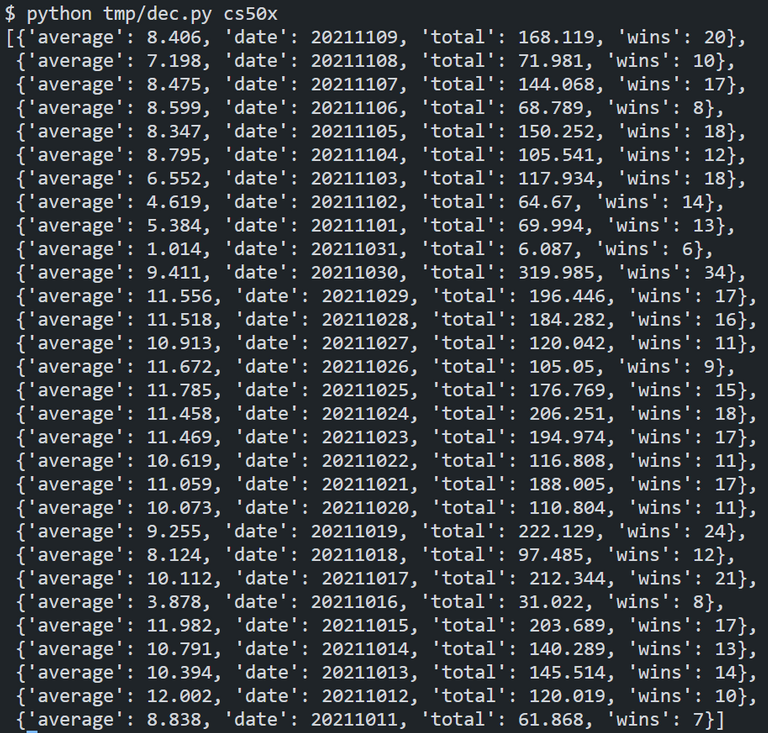# [Python] Code to calculate your total earned DEC per dayI wrote a Python code to calculate the total number of DEC won per day, the number of games won, and the average number of DEC won per game.

## Code

``````import sys
from datetime import datetime
from pprint import pprint
import requests

url = "https://api2.splinterlands.com/players/balance_history"
rows = requests.get(url + params).json()
d = []

for row in rows:
if row["type"] == "dec_reward":
dt = datetime.fromisoformat(row["created_date"].split('T'))
date_key = int(f"{dt.year}{str(dt.month).zfill(2)}{str(dt.day).zfill(2)}")
r = [r for r in d if r["date"] == date_key]
if r:
r["total"] = round(float(row["amount"]) + r["total"], 3)
r["wins"] += 1
r["average"] = round(r["total"] / r["wins"], 3)
else:
d.append({
"date": date_key,
"total": round(float(row["amount"]), 3),
"wins": 1
})
pprint(d)

``````

## Result0
0
0.000